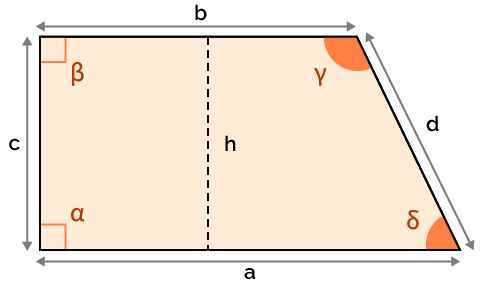# Right Trapezoid Calculator

Created by Łucja Zaborowska, MD, PhD candidate
Reviewed by Gabriela Diaz
Last updated: Feb 02, 2023

The right trapezoid calculator is indeed the right tool for computing all the properties of our favorite trapezoid.

We'll show you what's the difference between a 'typical' and a right trapezoid, and teach you how to calculate the slant of the right angle trapezoid. Follow the text for more!

## What's the right trapezoid?

A right trapezoid is a trapezoid with one of its legs perpendicular to both of the bases. In other words, it means that such a trapezoid must contain two right angles.

It should be our favorite type of trapezoids - it's much easier to calculate! It is because the height of the trapezoid is equal to one of its sides.

Don't you worry - our right trapezoid calculator is here to help you with all the necessary steps. 👌 We'll calculate:

• Right trapezoid area;
• Right trapezoid slant side;
• Right trapezoid height;
• Right trapezoid perimeter;
• Right trapezoid angles; and
• Right trapezoid median - available in the advanced mode.

💡 A rectangle is also a right trapezoid - it does satisfy the condition described above!

## How to calculate the slant of right angle trapezoid?

We can easily calculate the slant side of a right angle trapezoid using the Pythagorean theorem:

$d = \sqrt{(a-b)^2 + (c^2) }$

Where:

• d is the slant (long) side of a trapezoid;
• a is the longer base;
• b is the shorter base; and
• c is the short side (trapezoid's height).

## How to calculate the length of right trapezoid?

A right trapezoid is indeed special - its height is equal to the length of its shorter side. We can calculate it easily, using a modification of the Pythagorean theorem:

$b = \sqrt{ d^2 - (a-c)^2}$

Where:

• b is the shorter base;
• d is the slant (long) side of a trapezoid;
• a is the longer base; and
• c is the short side (trapezoid's height).

Happy with our right trapezoid calculator? Check out all the other amazing tools that'll help you compute all the trapezoid properties you may need:

## FAQ

### Does a trapezoid with one right angle exist?

No, it doesn't. A trapezoid may have two right angles or none at all.

Why is that so? 🤔

Two of the trapezoid bases must be parallel to each other. That's why, if one of the sides is at right angle (perpendicular) to one of the bases, it also must be perpendicular to the second one.

Łucja Zaborowska, MD, PhD candidatea (base)
in
b (base)
in
c (side/height)
in
d (slant side)
in
Area (A)
in²
Perimeter (P)
in
Angles
α
deg
β
deg
γ
deg
δ
deg
People also viewed…

### Car heat

The hot car calculator shows how fast a car's interior heats up during a summer day.

### Car vs. Bike

Everyone knows biking is fantastic, but only this Car vs. Bike Calculator turns biking hours into trees! 🌳

### Complementary angles

The complementary angles calculator determines the complementary angle to the one you input. You can also use this tool to check if two angles are complementary or not.